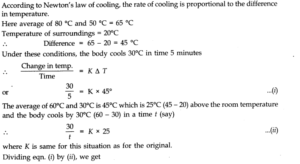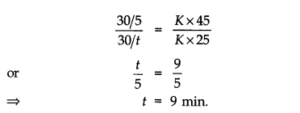# Chapter 11 – Thermal Properties of matter Questions and Answers: NCERT Solutions for Class 11 Physics

Class 11 Physics NCERT book solutions for Chapter 11 - Thermal Properties of matter Questions and Answers.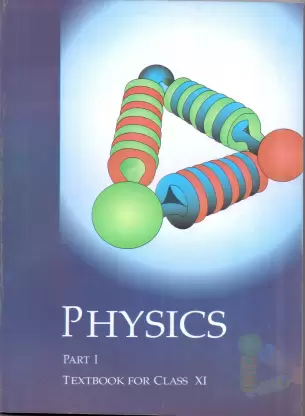## Question 11. 1. The triple points of neon and carbon dioxide are 24.57 K and 216.55 K respectively. Express these temperatures on the Celsius and Fahrenheit scales.

### Answer:The relation between kelvin scale and Celsius scale is TK– 273.15 =TC=> TC=TK– 273.15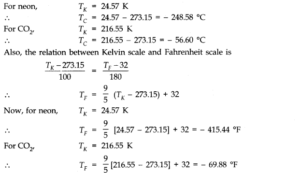## Question 11. 2. Two absolute scales A and B have triple points of water defined to be 200 A and 350 B. What is the relation between TAand TB?

### Answer:As we know, triple point of water on absolute scale = 273.16 K, Size of one degree of kelvin scale on absolute scale A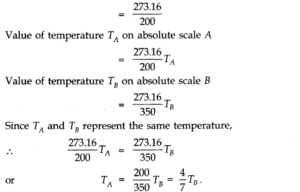## Question 11. 3. The-electrical resistance in ohms of a certain thermometer varies with temperature according to the approximate law: R = R0[1 + α (T – T0)]. The resistances is 101.6 Ωat the triple-point of water 273.16 K, and 165.5 Ωat the normal melting point of lead (600.5 K). What is the temperature when the resistance is 123.4 Ω ?

### Answer:Here, R0= 101.6 Ω; T0= 273.16 K Case (i) R1= 165.5 Ω; T1= 600.5 K, Case (ii) R2= 123.4 , T2= ? Using the relation R = R0[1 + α (T – T0)] Case (i) 165.5 = 101.6 [1 + α (600.5 – 273.16)]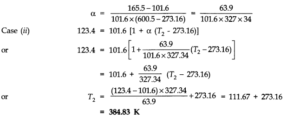## Question 11. 4. Answer the following: (a) The triple-point of water is a standard fixed point in modem thermometry. Why ? What is wrong in taking the melting point of ice and the boiling point of water as standard fixed points (as was originally done in the Celsius scale) ? (b) There were two fixed points in the original Celsius scale as mentioned above which were assigned the number 0 °C and 100 °C respectively. On the absolute scale, one of the fixed points is the triple-point of water, which on the Kelvin absolute scale is assigned the number273.16 K. What is the other fixed point on this (Kelvin) Scale ? (c) The absolute temperature (Kelvin scale) T is related to the temperature tcon the Celsius scaletc= T – 273.15 Why do we have 273.15 in this relation, and not 273.16 ? (d) What is the temperature of the triple-point of water on an absolute scale whose unit interval size is equal to that of the Fahrenheit scale ?

### Answer:(a) Triple point of water has a unique value i.e., 273.16 K. The melting point and boiling points of ice and water respectively do not have unique values and change with the change in pressure. (b) On Kelvin’s absolute scale, there is only one fixed point, namely, the triple-point of water and there is no other fixed point. (c) On Celsius scale 0 °C corresponds to the melting point of ice at normal pressure and the value of absolute temperature is 273.15 K. The temperature 273.16 K corresponds to the triple point of water. (d)The Fahrenheit scale and Absolute scale are related as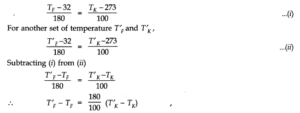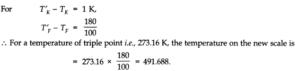## Question 11. 5. Two ideal gas thermometers A and B use oxygen and hydrogen respectively. The following observations are made: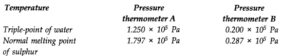## (a) What is the absolute temperature of normal melting point of sulphur as read by thermometers A and B ? (b) What do you think is the reason behind the slight difference in answers of thermometers A and B ? (The thermometers are not faulty). What further procedure is needed in the experiment to reduce the discrepancy between the two readings ?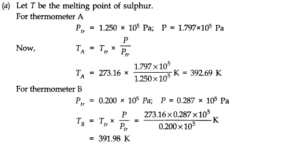## Question 11. 7. A large steel wheel is to befitted on to a shaft of the same material. At 27 °C, the outer diameter of the shaft is 8.70 cm and the diameter of the central hole in the wheel is, 8.69 cm. The shaft is cooled using ‘dry ice’. At what temperature of the shaft does the wheel slip on the shaft ? Assume coefficient of linear expansion of the steel to be constant over the required temperature range αsteel= 1-20 x 10-5K-1.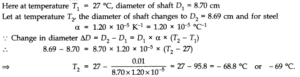## Question 11. 8. A hole is drilled in a copper sheet. The diameter of the hole is 4.24 cm at 27.0 °C. What is the change in the diameter of the hole when the sheet is heated to 227 °C ? Coefficient of linear expansion of copper = 1.70 x 10-5K-1.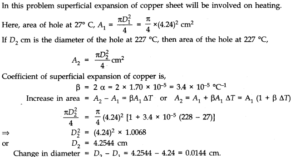## Question 11. 9. A brass wire 1.8 m long at 27 °C is held taut with little tension between two rigid supports. If the wire is cooled to a temperature of – 39 °C, what is the tension developed in the wire, if its diameter is 2.0 mm ? Co-efficient of linear expansion of brass = 2.0 x 10-5K-1; Young’s modulus of brass = 0.91 x 1011Pa

### Ans.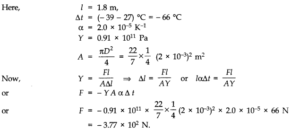## Question 11. 8. A hole is drilled in a copper sheet. The diameter of the hole is 4.24 cm at 27.0 °C. What is the change in the diameter of the hole when the sheet is heated to 227 °C ? Coefficient of linear expansion of copper = 1.70 x 10-5K-1.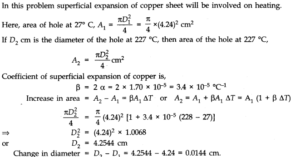## Question 11. 9. A brass wire 1.8 m long at 27 °C is held taut with little tension between two rigid supports. If the wire is cooled to a temperature of – 39 °C, what is the tension developed in the wire, if its diameter is 2.0 mm ? Co-efficient of linear expansion of brass = 2.0 x 10-5K-1; Young’s modulus of brass = 0.91 x 1011Pa

### Ans.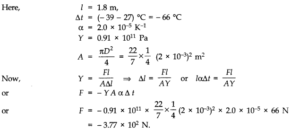## Question 11. 10. A brass rod of length 50 cm and diameter 3.0 mm is joined to a steel rod of the same length and diameter. What is the change in length of the combined rod at 250 °C, if the original lengths are at 40.0 °C ? Is there a ‘thermal stress’ developed at the junction ? The ends of the rod are free to expand (Co-efficient of linear expansion of brass = 2.0 x 10-5°C-1, steel = 1.2 x 10-5°C-3.

### Ans.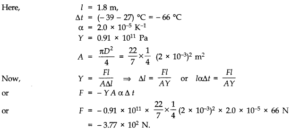## Question 11. 11. The coefficient of volume expansion of glycerine is 49 x 10-5K-1. What is the fractional change in its density for a 30 °C rise in temperature ?

### Ans.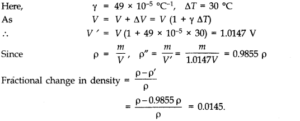## Question 11. 12. A 10 kW drilling machine is used to drill a bore in a small aluminium block of mass 8.0 kg. How much is the rise in temperature of the block in 2.5 minutes, assuming 50% of power is used up in heating the machine itself or lost to the surroundings? Specific heat of aluminium = 0.91 J g-1K-1.

### Answer:Power = 10 kW = 104W Mass, m=8.0 kg = 8 x 103g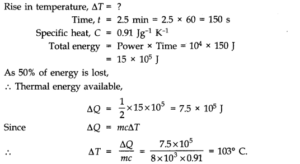## Question 11. 13. A copper block of mass 2.5 kg is heated in a furnace to a temperature of 500°C and then placed on a large ice block. What is the maximum amount of ice that can melt? Specific heat of copper is 0.39 Jg-1°C-1. Heat of fusion of water = 335 Jg-1.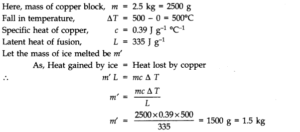## Question 11. 14. In an experiment on the specific heat of a metal, a 0.20 kg block of the metal at 150°C is dropped in a copper calorimeter (of water equivalent 0.025 kg) containing 150 cm3of water at 27 °C. The final temperature is 40° C. Compute the specific heat of the metal. If heat losses to the surroundings are not negligible, is your answer greater or smaller than the actual value for specific heat of the metal?

### Answer:Mass of metal block, m = 0.20 kg = 200 g Fall in the temperature of metal block, ΔT = (150 – 40) °C = 110 °C If C be the specific heat of metal, then heat lost by the metal block = 200 x C x 110 cal Volume of water = 150 cm3 mass of water = 150 g Increase in temperature of water = (40 – 27) °C = 13°C Heat gained by water = 150 x 13 cal Water equivalent of calorimeter, w = 0.025 kg = 25g Heat gained by calorimeter,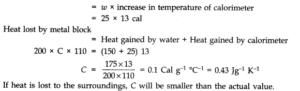## Question 11. 15. Given below are observations on molar specific heats at room temperature of some common gases.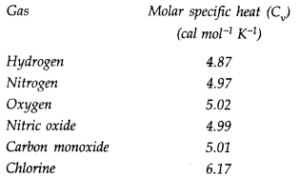## Question 11. 16. (a) At what temperature and pressure can the solid, liquid and vapour phases of CO2co-exist in equilibrium ? (b) What is the effect of decrease of pressure on the fusion and boiling point of CO2? (c) What are the critical temperature and pressure for CO2? What is their significance 1 (d) Is CO2solid, liquid or gas at (a) – 70 °C under 1 atm (b) – 60 °C under 10 atm (c) 15°C under 56 atm?

### Answer:(a) At the triple point, temperature = – 56.6 °C and pressure = 5.11 atm. (b) Both the boiling point and freezing point of CO2decrease if pressure decreases. (c) The critical temperature and pressure of CO2are 31.1°C and 73.0 atm respectively. Above this temperature, CO2will not liquefy/even if compressed to high pressures. (d) (i) The point (- 70 °C, 1.0 atm) lies in the vapour region. Hence, CO2is vapour at this point. (ii) The point (- 60 °C, 10 atm) lies in the solid region. Hence, CO2is solid at this point. (iii) The point (15 °C, 56 atm) lies in the liquid region. Hence, CO2is liquid at this point.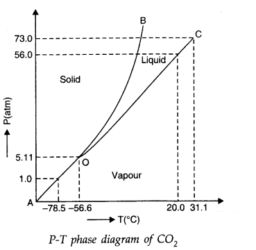## Question 11. 18. A child running a temperature of 101°F is given an antipyrin (i.e., a medicine that lowers fever) which causes an increase in the rate of evaporation of sweat from his body. If the fever is brought down to 98° F in 20 minutes, what is the average rate of extra evaporation caused by the drug ? Assume the evaporation mechanism to be the only way by which heat is lost. The mass of the child is 30 kg. The specific heat of human body is approximately the same as that of water, and latent heat of evaporation of water at that temperature is about 580 cal g-1.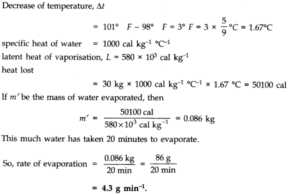## Question 11. 19. A ‘thermacole’ icebox is a cheap and efficient method for storing small quantities of cooked food in summer in particular. A cubical icebox of side 30 cm has a thickness of 5.0 cm. If 4.0 kg of ice is put in the box, estimate the amount of ice remaining after 6 h. The outside temperature is 45°C, and coefficient of thermal conductivity of thermacole is 0.01 Js-1m-1°C-1[Heat of fusion of water = 335 x 103J kg-1].

### Answer:Each side of the cubical box (having 6 faces) is 30 cm = 0.30 m. Therefore, the total surface area’ of the icebox exposed to outside air is A = 6 x (0.30 m)2= 0.54 m2. The thickness of the icebox is d = 5.0 cm = 0.05 m, time of exposure t = 6h = 6 x 3600 s and temperature difference T1– T2= 45°C – 0°C = 45°C. .•. Total heat entering the icebox in 6 h is given by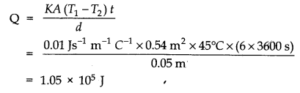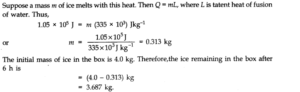## Question 11. 20. A brass boiler has a base area 0.15 m2and thickness 1.0 cm. It boils water at the rate of 6.0 kg/ min when placed on a gas stove. Estimate the temperature of the part of the flame in contact with the boiler. Thermal conductivity of brass = 109Js-1m-1K-1.(Heat of vaporization of water = 2256 x 103J kg-1)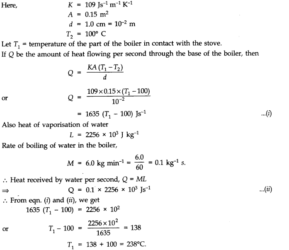## Question 11. 22. A body cools from 80 °C to 50°C in 5 minutes. Calculate the time it takes to cool from 60 °C to 30°C. The temperature of the surroundings is 20 °C.